ML Aggarwal Class 6 Solutions Chapter 10 Basic Geometrical Concept Ex 10.1 for ICSE Understanding Mathematics acts as the best resource during your learning and helps you score well in your exams.

## ML Aggarwal Class 6 Solutions for ICSE Maths Chapter 10 Basic Geometrical Concept Ex 10.1

Question 1.
How many lines can be drawn through a given point?
Solution: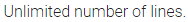Question 2.
How many lines can be drawn through two distinct given points?
Solution: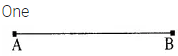Question 3.
How many lines can be drawn through three collinear points?
Solution: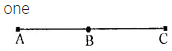Question 4.
Mark three non-collinear points A, B and C in your note-book. Draw lines through these points taking two at a time and name these lines. How many such different lines can be drawn?
Solution:Question 5.
Use the figure to name :
(i) Five point
(ii) Aline
(iii) Four rays
(iv) Five line segmentsSolution:Question 6.
Use the figure to name:(i) Line containing point E.
(ii) Line passing through A.
(iii) Line on which point O lies.
(iv) Two pairs of intersecting lines.
Solution: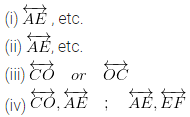Question 7.
From the given figure, write(i) collinear points
(ii) concurrent lines and their points of concurrence.
Solution: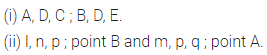Question 8.
In the given figure, write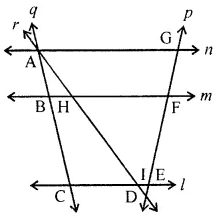(i) all pairs of parallel lines.
(ii) all pairs of intersecting lines,
(iii) concurrent lines.
(iv) collinear points.
Solution:Question 9.
Count the number of line segments drawn in each of the following figures and name them: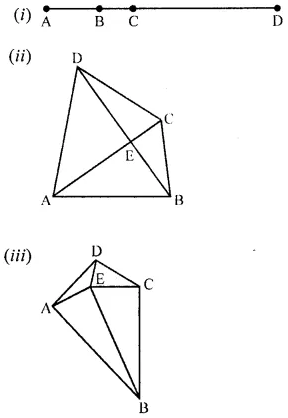Solution: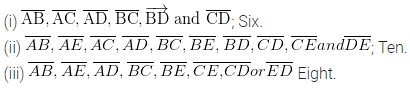Question 10.
(i) Name all the rays shown in the following figure whose initial points are A, B and C respectively.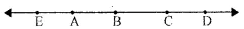(ii) Is ray AB different from ray AD?
(iii) Is ray CA different from ray CE?
(iv) Is ray BA different from ray CA?
(v) Is ray ED different from ray DE?
Solution:Question 11.
Consider the following figure of line $$\overleftrightarrow { MN }$$. Says whether following statements are true or false in context of the given figure.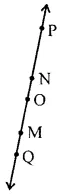(i) Q, M, O, N and P are points on the line
$$\overleftrightarrow { MN }$$.
(ii) M, O and N are points on a line segment
$$\overline{\mathrm{MN}}$$.
(iii) M and N are end points of line segment
$$\overline{\mathrm{MN}}$$ .
(iv) O and N are end points of line segment
$$\overline{\mathrm{OP}}$$.
(v) M is a point on the ray $$\overline{\mathrm{OP}}$$.
(vi) M is one of the end points of line segment
$$\overline{\mathrm{QO}}$$.
(vii) Ray $$\overrightarrow { OP }$$ is same as ray
$$\overrightarrow { OM }$$.
(viii)Ray $$\overrightarrow { OM }$$ is not opposite to ray
$$\overrightarrow { OP }$$.
(ix) Ray $$\overrightarrow { OP }$$ is different from ray
$$\overrightarrow { QP }$$.
(x) O is not an initial point of ray $$\overrightarrow { OP }$$.
(xi) N is the initial point of $$\overrightarrow { N }$$ and
$$\overrightarrow { NM }$$.
Solution: